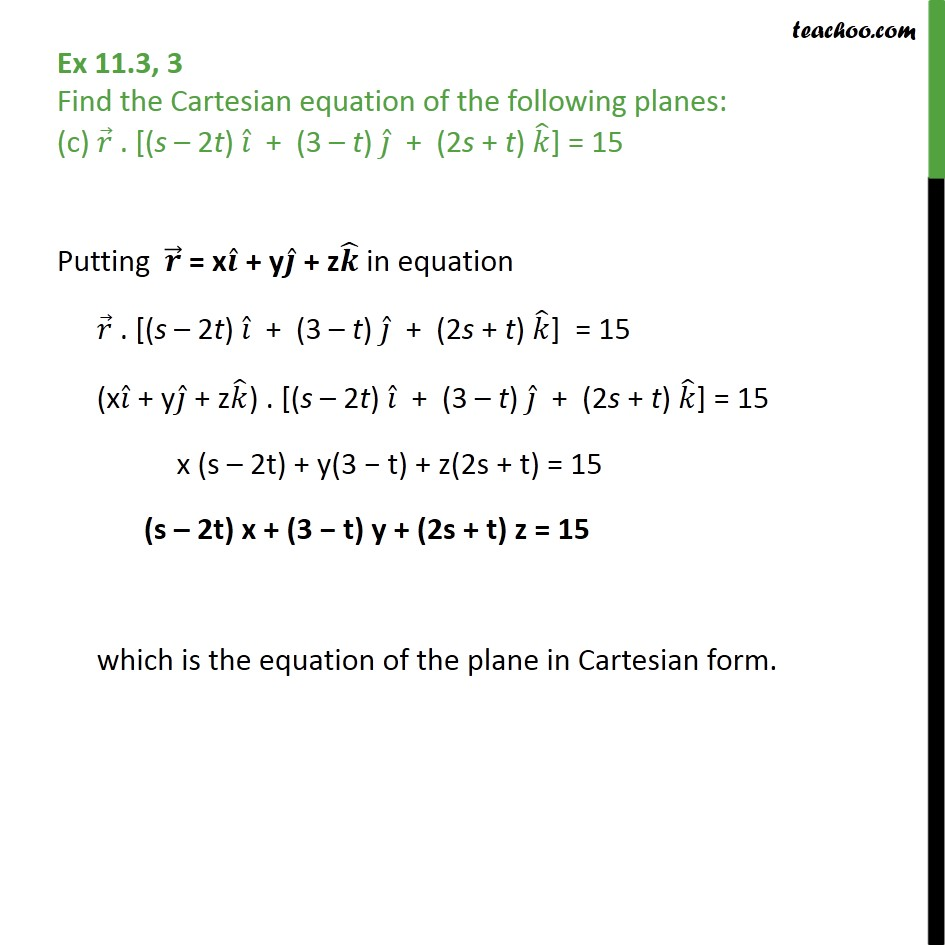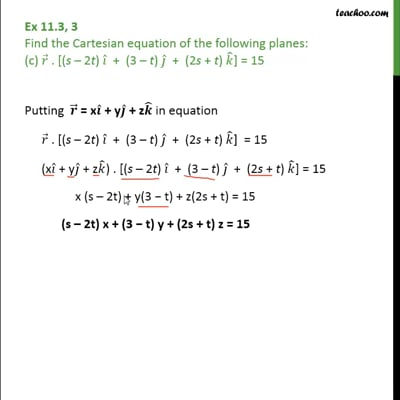Ex 11.3

Chapter 11 Class 12 Three Dimensional Geometry
Serial order wiseThis video is only available for Teachoo black users

Introducing your new favourite teacher - Teachoo Black, at only ₹83 per month

### Transcript

Ex 11.3, 3 Find the Cartesian equation of the following planes: (c) 𝑟﷯ . [(s – 2t) 𝑖﷯ + (3 – t) 𝑗﷯ + (2s + t) 𝑘﷯] = 15 Putting 𝒓﷯ = x 𝒊﷯ + y 𝒋﷯ + z 𝒌﷯ in equation 𝑟﷯ . [(s – 2t) 𝑖﷯ + (3 – t) 𝑗﷯ + (2s + t) 𝑘﷯] = 15 (x 𝑖﷯ + y 𝑗﷯ + z 𝑘﷯) . [(s – 2t) 𝑖﷯ + (3 – t) 𝑗﷯ + (2s + t) 𝑘﷯] = 15 x (s – 2t) + y(3 − t) + z(2s + t) = 15 (s – 2t) x + (3 − t) y + (2s + t) z = 15 which is the equation of the plane in Cartesian form.